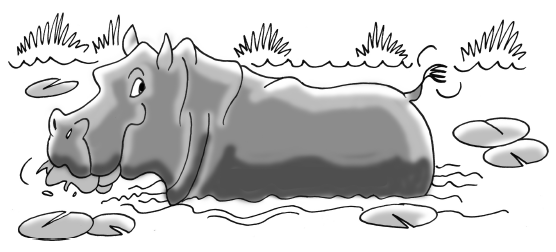### Home > GB8I > Chapter 5 Unit 6 > Lesson INT1: 5.2.1 > Problem5-55

5-55.

Harry the Hungry Hippo is munching on the lily pads in his pond. When he arrived at the pond, there were $20$ lily pads, but he is eating $4$ lily pads an hour. Heinrick the Hungrier Hippo found a better pond with $29$ lily pads! He eats $7$ lily pads every hour.1. If Harry and Heinrick start eating at the same time, when will their ponds have the same number of lily pads remaining?

Write an equation representing each hippo. Let $x=$ time (in hours) and $y=$ the number of lily pads. Note that the growth is negative.

Harry's equation is $y=20-4x$.

Set the two equations equal to each other and solve for $x$. They will have eaten the same number of lily pads when the equations for $y$ are equal.

$x=3$ hours
Don't forget to check your solution!

2. How many lily pads will be left in each pond at that time?

• Substitute $3$ back into one of the original equations and solve for $y$.